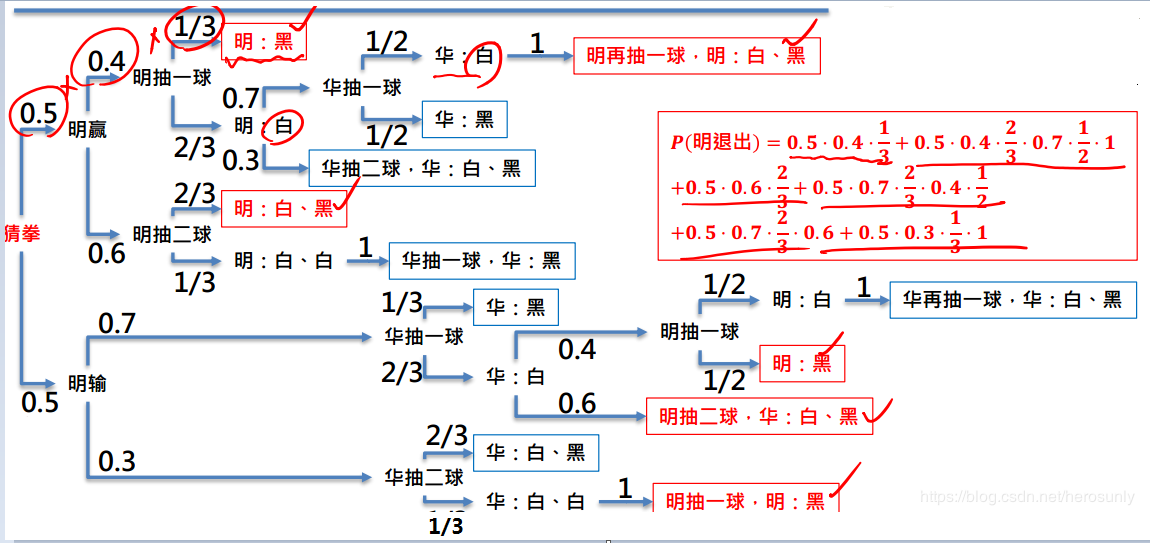2019-04-02 13:37:11 Yangchenju 阅读数 78
• ###### 【机器学习必知必会系列】高手必备数学教程-概率论

入门机器学习十分重要的三门数学课包括: 多元微积分, 线性代数, 概率统计. 机器学习&人工智能的高手必须懂数学，没有数学基础，只能做肤浅的事。姚老师使用 DeGroot<<概率统计>>作为讲解的基础, 对内部的知识点进行筛选, 将和机器学习相关性最大的内容讲解形成视频. 本视频是概率论的第一个阶段, 后续会继续更新第二阶段.

2380 人正在学习 去看看 姚青林

### 一、概率论基础

语言统计中常常会用到概率论知识，常用到的是概率、转移概率、条件概率。概率的概念这里不再描述。

#### 1.转移概率

转移概率是指从一个状态到另一个状态的概率，实际上是一种特殊的条件概率，即规定了邻接顺序的条件概率。
举例说明：
从“中国”转移到“人民”的概率为$P(W_2=“人民”|W_1=“中国”)$，可估计为“中国人民”的出现次数除以“中国”的出现次数。
类似地，从名词转移到动词的概率是$P(T_2=动词|T_1=名词)$，可估计为名词和动词相邻出现的次数除以名词出现的次数。

#### 2. 条件概率

条件概率不限于先后发生的事件。
举例说明：
$P(W_i=“设计”|T_i=“名词”)$表示在在某词为名词的条件下，其词形是“设计”的概率，当事件和作为条件的事件是同时发生的，应该看作是条件概率而不是转移概率。求这个条件概率，可以用语料库中作为名词出现的“设计”的出现次数除以所有名词出现的次数。
$P(W_i=名词|T_i=“设计”)$表示某词词形为“设计”的条件下，其词性为名词的概率，这是可以用语料库中名词“设计”的出现次数除以以任何词性出现词形为“设计”的次数。
条件可以用下面公式求：
$P(A|B)=N(AB)/N(B)$
即用事件AB发生的次数除以事件B发生的次数
如果已知事件AB、B的概率，可以用：
$P(A|B)=P(AB)/P(B)$

### 二、信息论基础

#### 1.信息熵

关于信息熵很多人会有一个模糊的概念，始终乱不清楚的内容，就算明白了也会很快忘掉：
事件发生的概率越低，说明不确定性越大，信息量就越大，信息熵就越大
信息熵是用来描述一个离散随机变量的不确定性的均值。计算公式如下：
$H(X)=H(P)=-\sum{p(x)log_2p(x)}$
它具备一下两个属性：

$H(X)>=0$
$H(X)=0$时，说明随机变量X是确定的，此时X没有信息可言。

#### 2. 联合熵

$(X,Y)$是一对离散型随机变量，其联合概率分布函数为$p(x,y)$，则联合熵：
$H(X,Y)=-\sum{\sum{p(x,y)log_2p(x,y)}}$
联合熵用来描述一对离散型随机变量平均所包含的信息量。

#### 3.条件熵

随机变量X已知的情况下，随机变量Y的条件熵：
$H(Y|X)=\sum{p(x)H(Y|X=x)}$
$=\sum{\sum{p(x,y)logp(y|x)}}$
熵的连锁规则：
$H(X,Y)=H(X)+H(Y|X)$
$H(X_1,X_2,...,X_n)=H(X_1)+H(X_2|X_1)+...+H(X_n|X_1,X_2,...,X_{n-1})$

#### 4.互信息

互信息是一种计算亮哥哥随机变量之间共有信息的度量。计算公式：
$I(X,Y)=H(X)-H(X|Y)$
$\sum{\sum{p(x,y)log{\frac{p(x,y)}{p(x)p(y)}}}}$
特点：

1. 当两个随机变量相互独立时，互信息为0
2. 当两个随机变量存在依赖关系时，互信息不为0

#### 5. 交叉熵

交叉熵用来衡量估计概率分布与真实分布之间的差异情况。
如果一个随机变量X~P(x)，q(x)时近似估计p(x)的概率分布，那么X和q(x)之间的交叉熵定义为：
$H(X,q)=-\sum{p(x)logq(x)}$

### 三、n元语法模型

一个语言模型通常构建为字符串s的概率分布p(s)，其中p(s)为s在某种语言中出现的概率。
对于一个有n个基元（字、词、短语、句等语言单位）构成的句子$s=w_1w_2w_3...w_d$，假设每个妓院只与前面相邻的n-1个基元有关，这样：
$p(s)=\prod{p(w_i|w_{i-n+1}...w_{i-1})}$
那么二元语法模型其实就是一个一阶马尔可夫链，所有$p(w_i|w_{i-1})$就组成了马尔可夫一阶转移概率矩阵。

### 四、语法模型的性能评价

交叉熵时用来评价语法模型性能的常用度量。语言L与其估计模型（n元语法模型）q的交叉熵为：
$H(L,q)=-lim_{x\to\infty}\frac{1}{n}\sum{p(x_{1n})logq(x_{1n})}$
假设语言L是稳态遍历的随机过程，即N趋于无穷大时，$p(x_{1n})$为常量。这时：
$H(L,q)=-lim_{n\to\infty}\frac{1}{n}logq(x_{1n})$
只要样本包含的词语数n足够大：
$H(L,q)\approx-\frac{1}{n}logq(x_{1n})$
在设计q时，交叉熵越小，模型就越接近真实的概率分布$p(x)$

图像处理用到概率论 相关内容

2019-12-17 16:34:10 qq_39378221 阅读数 79
• ###### 【机器学习必知必会系列】高手必备数学教程-概率论

入门机器学习十分重要的三门数学课包括: 多元微积分, 线性代数, 概率统计. 机器学习&人工智能的高手必须懂数学，没有数学基础，只能做肤浅的事。姚老师使用 DeGroot<<概率统计>>作为讲解的基础, 对内部的知识点进行筛选, 将和机器学习相关性最大的内容讲解形成视频. 本视频是概率论的第一个阶段, 后续会继续更新第二阶段.

2380 人正在学习 去看看 姚青林

# 概率论基础——概率论公理

概率论是研究随机现象数量规律的数学分支，是一门研究事情发生的可能性的学问。概率论的发展与赌博有关，一些学者研究了这些机会游戏中的简单问题。随着18、19世纪科学的发展，人们注意到在某些生物、物理和社会现象与机会游戏之间有某种相似性，从而由机会游戏起源的概率论被应用到这些领域中，极大地促进了概率论的发展。
我认为，理解概率论公理是一件十分重要的是，它是以往人们对概率问题描述的公理化，它是概率论的根，当概率有了明确的定义后，概率论才有飞速的发展，就像极限、无穷的定义后微积分的飞速发展一样。

## 1 样本空间和事件

### 1.1定义

这两个概念是概率论问题表示方法的基本组成元素。对于一个试验，其试验结果是无法肯定预测的，尽管试验之前无法得知试验结果，但是假设所有可能结果的集合是已知的。试验所有可能的结果构成的集合称为该试验的样本空间，记为$S$。这很好理解，例如抛一次硬币，其所有可能的结果就是正面和反面，$S=\{正面，反面\}$
事件（event）则是样本空间的任一子集，记为$E$，对于抛硬币的试验，令$E=\{正面\}$，那么该事件就是“抛出硬币为正面”。

### 1.2 相关定义

将定义符号化后，可以轻易地做出推导，这是文字表示难以达到的效果。现在对于同一个样本空间$S$的任意两个事件$E、F$，有如下几个新定义：

1. 事件$E\bigcup F$由以下结果组成：这些结果或在$E$中或在$F$中，或既在$E$中又在$F$中。即，事件$E$或事件$F$有一个发生，那么$E\bigcup F$就发生，称为$E$$F$
2. 事件$E\bigcap F$，或简写为$EF$，称为$E$$F$，它由$E$$F$的公共元素组成；
3. 不可能事件是指不可能发生的事件，记为$\varnothing$
4. 如果$EF=\varnothing$，则称$E$$F$是不相容的；
5. 对于任意事件$E$，定义事件$E$的补，表示包含在样本空间中，但不包含在$E$中的所有结果构成的事件，记为$E^c$
6. 如果$E$的所有都在$F$中，则称$E$包含于$F$，或$F$包含$E$，记为$E\subset F$$F \supset E。$

本质上都是集合的相关操作。足以见得集合论是概率论公理化的一个重要基础。

## 2 概率论公理

### 2.1 相对频率

一种定义事件发生概率的方法是利用事件发生的相对频率。定义：假设有一个样本空间为$S$的试验，它在相同的条件下可以重复进行，对于样本空间中的事件$E$，记$n(E)$$n$次重复试验中事件$E$发生的次数。那么，该事件发生的概率$P(E)$就定义如下：
$P(E)=\lim_{n\to \infty}\cfrac{n(E)}{n}$
即，定义概率$P(E)$$E$发生的次数占试验总次数的比例的极限，也即$E$发生频率的极限
这个定义看起来很符合我们现代人的直观感受，但是它却有很严重的缺陷——凭什么就说$\cfrac{n(E)}{n}$就一定会收敛到一个固定的常数呢？并且，如果再次进行同一个试验的重复试验，怎么就能保证它还会收敛到相同的常数呢？这种缺陷的存在是不能够进行严谨的数学推理的，如何定义概率并把概率论建立在严谨的逻辑上是概率论发展的一个困难，直到20世纪初完成的勒贝格测度与积分理论及随后发展的抽象测度和积分理论，为概率公理体系的建立奠定了基础。在这个背景下，苏联数学家柯尔莫哥洛夫1933年在他的《概率论基础》一书中第一次给出了概率的测度论的定义和一套严密的公理体系。他的公理化方法成为现代概率论的基础，使概率论成为严谨的数学分支，对概率论的迅速发展起了积极的作用。

### 2.2 概率论公理

柯尔莫哥洛夫提出的概率论公理成为了概率论发展的根，有了严谨的定义后，才有之后的发展。假设某个试验的样本空间为$S$，对应于其中任一事件$E$，定义一个实数$P(E)$，它满足以下3个公理：

• 公理1：非负性，$0\le P(E)\le 1$
• 公理2：规范性，$P(S)=1$
• 公理3：可列（完全）可加性，对任一列互不相容的事件$E_1,E_2,\cdots$，(符号的表示为如果$i\ne j$，则$E_iE_j=\varnothing$)，有:
$P(\bigcup_{i=1}^\infty E_i)=\sum_{i=1}^\infty P(E_i)$

### 2.3 由公理得到的命题

有了公理，也就有了树根，根据公理我们会得到几个命题：

• $1=P(E)+P(E^c)$，一个事件不发生的概率等于1减去一个它发生的概率；
• 如果$E\subset F$，那么$P(E)\le P(F)$
• $P(E\bigcup F)=P(E)+P(F)-P(EF)$
• 容斥恒等式:
$P(E_1\bigcup E_2\bigcup \cdots \bigcup E_n)=\sum_{i=1}^nP(E_i)-\sum_{i_1\lt i_2}P(E_{i_1}E_{i_2})+\cdots+\\ (-1)^{r+1}\sum_{i_1\lt i_2\lt \cdots \lt i_r}P(E_{i_1}E_{i_2}\cdots E_{i_r}) +\cdots+(-1)^{n+1}P(E_1E_2\cdots E_r)$
其中，$\sum_{i_1\lt i_2\lt \cdots \lt i_r}P(E_{i_1}E_{i_2}\cdots E_{i_r})$表示对一切下标集合$\{i_1,i_2,\cdots,i_r\}$所对应的值求和，和项一共包含$\begin{pmatrix} n\\ r\end{pmatrix}$项。

## 3 等可能结果的样本空间

对于一个试验，我们一般很自然地会假设，样本空间中的所有结果发生的可能性都是一样的。这是传统概率也叫拉普拉斯概率，如果一个随机试验所包含的单位事件是有限的，且每个单位事件发生的可能性均相等，则这个随机试验叫做拉普拉斯试验。传统概率在实践中被广泛应用于确定事件的概率值，其理论根据是：如果没有足够的论据来证明一个事件的概率大于另一个事件的概率，那么可以认为这两个事件的概率值相等
考虑一个试验，其样本空间$S$是有限集，设$S=\{1,2,\cdots N\}$，我们会根据传统概率来确定每一个单独结果的概率：
$P(\{1\})=P(\{2\})=\cdots=P(\{N\})$
根据公理2、3上式意味着：
$P(\{i\})=\cfrac{1}{N}$
再根据公理3，对于任何事件$E$，事件发生的概率$P(E)$为：
$P(E)=\cfrac{E中的结果数}{S中的结果数}$

## 4 概率是确信程度的度量

概率可以是人们对自己的说法的确信程度的一种度量。经常被称为主观概率

参考资料：
《概率论基础教程》Sheldon M.Ross
百度百科——概率论

图像处理用到概率论 相关内容

2016-09-08 01:01:35 Lee_zix 阅读数 1340
• ###### 【机器学习必知必会系列】高手必备数学教程-概率论

入门机器学习十分重要的三门数学课包括: 多元微积分, 线性代数, 概率统计. 机器学习&人工智能的高手必须懂数学，没有数学基础，只能做肤浅的事。姚老师使用 DeGroot<<概率统计>>作为讲解的基础, 对内部的知识点进行筛选, 将和机器学习相关性最大的内容讲解形成视频. 本视频是概率论的第一个阶段, 后续会继续更新第二阶段.

2380 人正在学习 去看看 姚青林

概率论，那我们先来说说什么是概率

Probability:Probability is the measure of the likelihood that an will occur.
这里的event其实是指我们的统计实验（Statistic Ecperiment）的结果。
而我们的概率就是用来定量的衡量我们的实验结果出现的可能性的。用数学的角度看，我们的统计实验产生了一系列的样本点，由这些样本点组成了我们的样本空间，我们的event，可以看成是这些样本空间的真子集。那么我们的概率，则可以定义为从样本空间的真子集到实数集的映射。

P:2ΩR$P:{2} ^ {\Omega} \rightarrow \mathbb{R}$

概率有以下特性:

1. P()=0,P(Ω)=1$P(\varnothing)=0, P(\Omega)=1$
2. 可数可加性：
AkΩ,AiAj=,(ij)$A_k\subset \Omega , A_i \cap A_j = \varnothing,(i \neq j)$
P(Ak)=Ai$P(\bigcup A_k) = \sum{A_i}$

随机变量：is a variable whose value is subject to variations due to chance。

随机变量并不是随机的，概率论中的随机性只是体现在从统计实验产生样本点的过程中，而随机变量，他本质上是一个确定的，有样本空间映射到实数的函数，他要解决的是样本点的量化问题。

随机变量本身是不具有随机性的

样本空间 Ω={w1,w2...wn...}$\Omega = \{w_1,w_2...w_n...\}$
随机变量X$X$为从Ω$\Omega$到实数集R$\mathbb{R}$的函数，记为：
XΩR$X ： \Omega \rightarrow \mathbb{R}$
我们常使用P(X=xk)=Pk$P(X=x_k)=P_k$为随机变量X$X$ 的概率分布。我们需要仔细看下这个符号的含义，概率P是定义在样本空间的子集上的，那么，X=xk$X=x_k$对应了一个什么样的样本空间子集呢？我们假设样本空间的子集wk$w_k$满足P({wk})=Pk$P(\{w_k\}) = P_k$
X=Xk$X=X_k$的含义是是在样本空间中寻找满足P({wk})=Pk$P(\{w_k\}) = P_k$的样本点集合w$w$，，该样本点集合对应的随机变量函数的值记为：
P(X=xk)=P(w:X(w)=Xk)=Pk$P(X=x_k)=P({w:X(w)=X_k}) = P_k$

## Discrote 离散随机变量

1.$\rm1.$布努力分布
用最简单的抛硬币为例，
样本空间 Ω={}$\Omega=\{“正”，“反”\}$，随机变量为定义在{“正”，“反”}映射到{0，1}上的函数，其中P(X=1)=p$P(X=1) = p$, P(X=0)=1p$P(X=0)=1-p$

1.$\rm1.$二项分布
已射击问题为例，打了n枪，每一枪命中的概率为p，n枪中有k枪命中的概率。
X B(n,p)$X~B(n,p)$ P(X=k)=(nk)pk(1p)nk$P(X=k)=\binom{n}{k}p^k(1-p)^{n-k}$, 这个概率的得出是在假设每一枪的射击事件是独立的前提的下得，然而现实中很明显每一枪的射击都是互相干扰的，独立性的判断，是概率论中最难的问题之一。我们都知道，如果事件A 和事件 B满足 P(AB)=P(A)P(B)$P(A\cap B)=P(A)*P(B)$,则两个事件相互独立。

3.$\rm3.$几何分布
已射击问题为例，表示从开始射击到第一次命中的概率
P(X=k)=(1p)k1p$P(X=k) = (1-p)^{k-1}*p$
几何分布有一个很重要的性质，无记忆性(Memoryless):
P(X>n+k|x>k)=P(x>n)$P(X>n+k|x>k)=P(x>n)$
这里我们引出了一种新的概率，条件概率：
P(A|B)=P(AB)/P(B)$P(A|B) = P(A \cap B)/P(B)$
条件概率是一类很特殊的概率，因为其样本空间不同于P(A)$P(A)$或者P(B)$P(B)$的样本空间，其样本空间缩小到事件B对应的样本空间的子集上，当且仅当 事件A和事件B相互独立时，P(A|B)=P(A)$P(A|B) = P(A)$

对于几何分布
P(X>k)=i=kP(x=i)=i=k(1p)i1p$P(X>k)=\sum_{i=k}^ \infty P(x=i)=\sum_{i=k}^ \infty (1-p)^{i-1}*p$

4.$\rm4.$泊松分布

P(X=k)=λkk!exp(λ)$P(X=k)= \frac{ \lambda^k} {k!} exp(- \lambda)$
泊松分布是在二项分布在n>$n->\infty$,p>0$p->0$，同时np=λ$np=\lambda$的极限。
证明：

泊松分布表示命中率极低同时尝试次数极多的射击过程，形象的说，泊松分布描述的是一个等待行为，同时，这在计算机中可以描述为一种网络行为，同时在排队论中也有重要的作用

至于为什么很多现象都服从泊松分布，不妨可以从二项分布的角度去考虑。
假如一个独立事件发生的概率是p，那么做n次独立实验，最后的结果服从二项分布（可以从抛硬币的问题中理解）
而在日常生活中，很多情况下都是独立事件，比如一段路口一天内发生车祸的概率p非常小，但如果你观察很多天，由于每两天之间的车流几乎是相互独立的，所以可以近似认为这个路口发生车祸的情况服从泊松分布。

泊松分布是指某段连续的时间内某件事情发生的次数，而且“某件事情”发生所用的时间是可以忽略的。例如，在五分钟内，电子元件遭受脉冲的次数，就服从于泊松分布。

假如你把“连续的时间”分割成无数小份，那么每个小份之间都是相互独立的。在每个很小的时间区间内，电子元件都有可能“遭受到脉冲”或者“没有遭受到脉冲”，这就可以被认为是一个p很小的二项分布。而因为“连续的时间”被分割成无穷多份，因此n(试验次数)很大。所以，泊松分布可以认为是二项分布的一种极限形式。

## Continuous 连续随机变量

之前讨论的样本空间都是离散的一个个的样本点，当我们的样本空间Ω=R$\Omega=\mathbb{R}$时，我们之前定义的单点的概率值P(w)=0$P({w})=0$

图像处理用到概率论 相关内容

2019-12-04 15:26:17 herosunly 阅读数 29
• ###### 【机器学习必知必会系列】高手必备数学教程-概率论

入门机器学习十分重要的三门数学课包括: 多元微积分, 线性代数, 概率统计. 机器学习&人工智能的高手必须懂数学，没有数学基础，只能做肤浅的事。姚老师使用 DeGroot<<概率统计>>作为讲解的基础, 对内部的知识点进行筛选, 将和机器学习相关性最大的内容讲解形成视频. 本视频是概率论的第一个阶段, 后续会继续更新第二阶段.

2380 人正在学习 去看看 姚青林

# 0. 基本概念

实验(experiment)包括了步骤(procedures)、概率模型(model)、观察(observation)。
结果是实验中可能出现的结果(outcome)。
样本空间是实验所有可能结果的集合。（Sample Space）简称S。
事件代表的是对实验结果的某种描述，也可以看成是结果的集合，是样本空间的子集。
概率就是实验结果符合某事件描述的机会有多大。

事件空间的本质是set of set，样本空间属于事件空间。概率是个函数，是从事件空间到[0,1]的映射。

# 1. 概率计算

## 1.1 图解复杂概率问题

范例：兄弟情。明、华兄弟情笃。故决定一人放弃追求小美以免伤情谊。于罐中放入两白球、一黑球。游戏规则如下：猜拳决定谁先，之后轮流罐中取球；每次可取一至二球，直至有人抽中黑球为止(不放回取球)。抽中黑者退出追求。

已知猜拳输赢机率为0.5，每次明取球取一颗之机率为0.4，取两颗机率为0.6 。每次华取球取一颗之机率为0.7，取两颗机率为0.3。问最后小明退出追求之机率为？# 2. 随机变量

随机变量不是变量，而是实验结果的函数。它是把实验结果进行数字化的函数。$X:S\rightarrow R$

## 2.1 随机变量的种类

随机变量分为离散型随机变量和连续性随机变量。离散型随机变量指的是随机变量的值是有限个或者可数的无穷多个。

### 2.1.1 可数和不可数

一个集合若是不可数的，这代表它包含的东西是无法可以一个个被数的。不管用什么方法数它里面的东西，它里面一定有一样东西是你没数到的！

第 N位数字定为“9 −第 N 个被数数字的第 N位数字

# 3. 概率论和数理统计的关系是什么？

概率论是数理统计的基础，而数理统计是概率论的应用。数理统计是通过采集数据、数据分析、得出尽可能正确的结论。其中数据分析指的是选择模型和参数估计。而选择模型和参数估计就会用到概率论。

## 3.1 为什么得到的是尽可能正确的结论

采集数据本质上是对总体进行采样，只有数据量解决无穷大才能得到正确的结论。而样本数量有限，就会使得结论有误差，但我们要得到尽可能正确的结论（前提是每个样本被采样的概率相等）。

得到结论后，我们需要对结论进行进一步判断，接受或者拒绝该结论。但可能会出现两个问题，以灯泡寿命问题为例，得到了样本平均值$\overline { X }$，将$\overline {X}$和指定数$l$进行比较，从而接收或者拒绝这批灯泡。

但可能会出现两个问题，在进行假设检验时提出原假设和备择假设，原假设实际上是正确的，但我们做出的决定是拒绝原假设，此类错误称为第一类错误。原假设实际上是不正确的，但是我们却做出了接受原假设的决定，此类错误称为第二类错误。

# 4.一维随机变量

## 4.1 离散型随机变量

设某事件A在一次试验中发生的概率为$p$，现把这个试验独立地重复$n$次，$X$$n$次试验中$A$发生的次数，则$X$可取$0,1,\dots n$等值。为确定其概率分布，考虑事件$\{X=i\}$。要这个事件发生，必须在这$n$次试验的原始记录
$AA\overline{A}\dots\overline{A}A\overline{A}$

中，有$i$$A$$n-i$$\overline{A}$，每个$A$有概率$p$，而每个$\overline{A}$有概率$1-p$

图像处理用到概率论 相关内容

2016-07-13 12:14:09 Shingle_ 阅读数 905
• ###### 【机器学习必知必会系列】高手必备数学教程-概率论

入门机器学习十分重要的三门数学课包括: 多元微积分, 线性代数, 概率统计. 机器学习&人工智能的高手必须懂数学，没有数学基础，只能做肤浅的事。姚老师使用 DeGroot<<概率统计>>作为讲解的基础, 对内部的知识点进行筛选, 将和机器学习相关性最大的内容讲解形成视频. 本视频是概率论的第一个阶段, 后续会继续更新第二阶段.

2380 人正在学习 去看看 姚青林

对于从事统计自然语言处理来说，了解概率论、信息论以及语言学知识都是很有必要的。
下面内容主要介绍了在统计自然语言处理中需要了解的概率论基础

## 概率

如果P(A)作为事件A的概率，Ω是试验的样本空间，则概率函数满足下面三条公理：

• 非负性 P(A) >= 0
• 规范性 P(Ω) = 1
• 可列可加性：对于不相交的集合Aj ∈F## 条件概率和独立性

假设事件B的概率已知，那么事件A发生的条件概率为（P(B) > 0）:在统计自然语言处理中，上面那个链式法则很有用处，比如推导马尔可夫模型的性质。

## 贝叶斯定理

由条件概率和链式规则推得：右边的分母P(A)可以看作是归一化常数，以保证其满足概率函数的性质。
如果我们感兴趣的仅仅是事件发生的相对可能性，这时可以忽略分母：## 随机变量

设X为一离散型随机变量，其全部可能的值为{a1,a2,···}。那么：
pi = P(X = ai), i = 1, 2, ····
称为X的概率函数。
P(X <= x) = F(x), x∈R
称为X的分布函数。## 期望和方差## 联合分布和条件分布

设两个离散随机变量X和Y，它们的联合密度函数可以写为：描述其中单个随机变量的概率密度函数称为边缘密度函数：## 标准分布

### 离散分布函数：二项分布

重复一个只有两种输出的实验，并且每次实验之间相互独立时，我们认为实验结果服从二项分布（例如抛硬币实验）。
在自然语言处理中，语料库中的句子间肯定不是完全相互独立的。但是为了简化问题的复杂性，我们通常可能会做独立性假设，假设一个句子的出现独立于它前面的其他句子，近似认为它们服从二项分布。当实验有两个以上结果时，二项分布问题就转化为多项式分布（multi-nomial distribution）。

### 连续分布函数：正态分布图像处理用到概率论 相关内容

阅读数 6839

博文 来自： olizxq

阅读数 2612

博文 来自： HuJinzhang

阅读数 44

博文 来自： qq_41262681

阅读数 377

博文 来自： dongrixinyu

阅读数 5142

博文 来自： angelazy
没有更多推荐了，返回首页